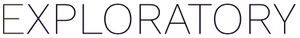# Color bar chart by continuous variable

#1

Hi - I just want to color each entire bar by the sum of sales, not sure how to do this as currently each bar is getting multiple shades of color. Thanks!

Basically, the equivalent of this code:

``````store %>%
group_by(yearmo = floor_date(order_date,'month')) %>%
summarise(sum_sales = sum(sales)) %>%
ggplot(aes(yearmo,sum_sales,fill=sum_sales))+
geom_col()+
scale_fill_distiller(palette = 'Blues',direction=1)

``````

#2

Hi @Bruce_T

I do not know whether this is the best solution, but it is possible to generate a graph equivalent of what you’re achieving with script R.

Aggregate the data by year/month before creating a bar chart.

After create a bar chart and color in the sum of the amounts.